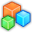# Microsoft Small BasicProgram Listing: RQS498
CRLF = Text.GetCharacter(13) + Text.GetCharacter(10)
gw = 598
gh = 428
GraphicsWindow.Width = gw
GraphicsWindow.Height = gh
GraphicsWindow.BrushColor = "Black"
GraphicsWindow.FontName = "Trebuchet MS"
instruction = "A : Angle = 45" + CRLF
instruction = instruction + "X : X = 100" + CRLF
instruction = instruction + "Y : Y = 100" + CRLF
instruction = instruction + "M : Move(100)" + CRLF
instruction = instruction + "2 : MoveTo(200, 200)" + CRLF
instruction = instruction + "T : Turn(180)" + CRLF
instruction = instruction + "L : TurnLeft()" + CRLF
instruction = instruction + "R : TurnRight()"
GraphicsWindow.DrawBoundText(10, 10, gw - 20, instruction)
Turtle.Show()
GraphicsWindow.KeyDown = OnKeyDown
Sub OnKeyDown
key = GraphicsWindow.LastKey
GraphicsWindow.Title = key
If key = "A" Then
Turtle.Angle = 45
ElseIf key = "X" Then
Turtle.X = 100
ElseIf key = "Y" Then
Turtle.Y = 100
ElseIf key = "M" Then
Turtle.Move(100)
ElseIf key = "D2" Then
Turtle.MoveTo(200, 200)
ElseIf key = "T" Then
Turtle.Turn(180)
ElseIf key = "L" Then
Turtle.TurnLeft()
ElseIf key = "R" Then
Turtle.TurnRight()
EndIf
EndSub